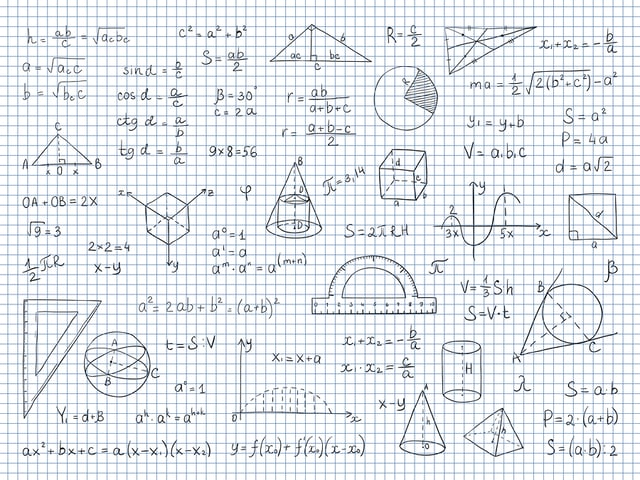# Formulas for Addressing the Additional Topics in Math Questions on the SAT® Exam

How to address the Additional Topics in Math questions on the SAT® Exam? First, you have to work on your spatial skills, since most of the questions will be related to geometry. You need to have the skills of visualizing geometric shapes and working with the appropriate formulas for each shape.

That’s where we can help you! In the following formula chart, you’ll find the essential formulas you’ll need for acing those Additional Topics in Math questions! Use them to solve our practice test at Union Test Prep, and prepare for the SAT® Test!

Also, practice using formula charts that cover the other three areas covered by the SAT® Math Test:

For now, try these formulas out in Additional Topics questions:

Category Formula Symbols Comment
Topics in
Math
$$P=4 \cdot s$$ P = Perimeter of a square
s = Side length

Topics in
Math
$$P=(2 \cdot l)+(2 \cdot w)$$ P = Perimeter of a rectangle
l = Length
w = Width

Topics in
Math
$$P=s_1 + s_2 + s_3$$ P = Perimeter of a triangle
$$s_n$$ = Side length

Topics in
Math
$$C=2 \cdot \pi \cdot r = \pi \cdot d$$ C = Circumference (perimeter) of a circle
d = Diameter
$$\pi \approx$$ 3.14

Topics in
Math
$$S=r\theta$$ S = Arc length
$$\Theta$$ = Central angle (in radians)

Topics in
Math
$$A=s^2$$ A = Area of a square
s = Side length

Topics in
Math
$$A = l \cdot w$$ A = Area of a rectangle
l = Length
w = Width

Topics in
Math
$$A= \frac{1}{2} \cdot b \cdot h$$ A = Area of a triangle
b = Base
h = Height (altitude)

Topics in
Math
$$A = \pi r^2$$ A = Area of a circle

Topics in
Math
$$A= h \cdot \dfrac{(b_1 + b_2)}{2}$$ A = Area of a trapezoid
$$b_n$$ = Base n
h = Height (altitude)

Topics in
Math
$$V=s^3$$ V = Volume of a cube
s = side length

Topics in
Math
$$V = l \cdot w \cdot h$$ V = Volume of a rectangular prism
l = Length
w = Width
h = Height

Topics in
Math
$$V = \frac{4}{3} \cdot \pi \cdot r^3$$ V = Volume of a sphere

Topics in
Math
$$V = \pi \cdot r^2 \cdot h$$ V = Volume of a cylinder
h = height

Topics in
Math
$$V= \frac{1}{3} \cdot \pi \cdot r^2 \cdot h$$ V = Volume of a cone
h = Height

Topics in
Math
$$V= \frac{1}{3} \cdot l \cdot w \cdot h$$ V = Volume of a pyramid
l = Length
w = Width
h = Height

Topics in
Math
$$d = \sqrt{(y_2-y_1)^2 + (x_2-x_1)^2}$$ d = Distance between two points
$$y_n$$ = y value at point n
$$x_n$$ = x value at point n

Topics in
Math
$$a^2 + b^2 = c^2$$ a, b = Legs of a right triangle
c = Hypotenuse of a right triangle
Pythagoras’ Theorem
Topics in
Math
$$(x-h)^2 + (y-k)^2 = r^2$$ (h, k) = Center of a circle
Standard form of
a circle
Topics in
Math
$$x^2+y^2+Ax+By+C=0$$ x, y = variables
A, B, C = constants
General form of a circle
Topics in
Math
$$sin^2 \theta + cos^2 \theta = 1$$ $$\theta$$ = Any angle
Topics in
Math
$$sin \ 2\theta = 2 \cdot sin \theta \cdot cos \theta$$ $$\theta$$ = Any angle
Topics in
Math
$$cos\ 2\theta = cos^2\theta - sin^2\theta = 2\ cos^2\theta-1$$ $$\theta$$ = Any angle
Topics in
Math
$$tan\ 2\theta = \dfrac{2\ tan \theta}{1-tan^2 \theta}$$ $$\theta$$ = Any angle
Topics in
Math
$$(a+bi) + (c+di) = (a+c) + (b+d)i$$ a, b, c, d = Constants
$$i = \sqrt{-1}$$
numbers
Topics in
Math
$$(a+bi) \cdot (c+di) = [(a \cdot c)+(a \cdot d)i] +[(b \cdot c)i+(b \cdot d)(-1)]$$ a, b, c, d = Constants
$$i = \sqrt{-1}$$
Multiplication of
complex numbers
Topics in
Math
$$\dfrac{a+bi}{c+di} = \dfrac{ac+bd}{c^2+d^2} + i\dfrac{bc-ad}{c^2+d^2}$$ a, b, c, d = Constants
$$i = \sqrt{-1}$$
Division of complex
numbers

### Formulas with Graphic Reference:$sin\ \theta = \dfrac {opposite}{hypotenuse} = \dfrac{1}{csc\ \theta}$ $cos\ \theta = \dfrac {adjacent}{hypotenuse} = \dfrac{1}{csc\ \theta}$ $tan\ \theta = \dfrac {opposite}{adjacent} = \dfrac{1}{csc\ \theta}$$\dfrac{a}{sin\ A} = \dfrac{b}{sin\ B} =\dfrac{c}{sin\ C}$ $a^2 = b^2 + c^2 - 2\ bc\ cos\ A$ $b^2 = a^2 + c^2 - 2\ ac\ cos\ B$ $c^2 = a^2 + b^2 - 2\ ab\ cos\ C$